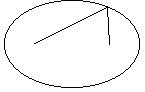Come new school year, wide spread of computers, networks, World Wide Web notwithstanding, student are going to use scissors, rulers, compass and other (analog) gadgets for class work, home assignments and ultimately learn and achieve not necessarily academic goals. There is indeed a great variety of analog devices available that often provide an unexpected and edifying experience. Some could be simulated on a computer with added flexibility and ease of access. One is presented below.

Now, I assume that everyone remembers a simple property of right-angled triangles - a median from the right angle to the hypotenuse equals one half of the latter. This fact leads to the way of drawing circles illustrated by the applet below. To see how it works, note that the red segment can be dragged with the endpoint on the horizontal line. The mid point of the segment will then trace a circle. (Do not forget to check/uncheck the Trace box.) Do you see why?

17 October 2015, Created with GeoGebraThe gadget takes you one step further. The midpoint can be also dragged along the segment. Shift it a little and then slide the segment again. What curve do you obtain this time? It's an ellipse. Using Analytic Geometry, the proof is fairly straightforward. If the sliding segment is of length a + b, and the drawing point is at the distance a from one end and the distance b from the other, then the equation of the curve so drawn will be either x²/a² + y²/b² = 1 or x²/b² + y²/a² = 1.

The applet actually simulates a carpenter's tool known as trammel. As [Dorrie, p. 215] describes it, "A trammel consistsof a cross with two grooves at right angles to each other in which two sliding pins A and B move. The pins are fixed to a beam to which at some point a moveable pencil M can be attached. Wehn the pins slide in the grooves the pencil describes an ellipse with the half axis AM and BM." Trammel is an ancient device and is often referred to as a trammel of Archimedes.

As a matter of fact, ellipse would be obtained even if the two axis were not perpendicular. Moreover, the drawing point M need not be collinear with the pins A and B but only rigidly connect to them, like the third vertex of a fixed triangle. Drawing ellipses in this manner is credited to F. van Schooten, a seventeenth century Ducth mathematician.

Although simple, this gadget furnishes an opportunity for several exercises:

• Trigonometry: triangle solving
• Conic sections: gathering all ellipse parameters from an equation
• Analytic Geometry: straight line equation r = r0 + t·(r - r0) which is useful in locating the drawing point### A reminder

In a circle, an inscribed right angle subtends the circle's diameter. So that the median from the right angle equals the radius of the circle whereas the hypotenuse is equal to its diameter.Paul Kunkel from Washington describes a curious device - planimeter - that has been widely used until about 20-30 yeas ago for area estimates.## References

1. H. Dorrie, 100 Great Problems of Elementary Mathematics: Their History and Solution, NewYork, Dover, 1989.
2. V. Gutenmacher, N. Vasilyev, Lines and Curves: A Practical Geometry Handbook , Birkhauser; 1 edition (July 23, 2004)### Conic Sections > Ellipse